# Synchronous Detectors Facilitate Precision Low-Level Measurements

### Introduction

Synchronous detectors can extract small signals, buried in the noise floor, to measure properties such as very small resistance, light absorption or reflection against bright backgrounds, or strain in the presence of high noise levels.

In many systems, the noise increases as the frequency approaches zero. For example, op amps have 1/f noise and light measurement is subject to noise from changing ambient light conditions. Moving the measurement away from the low-frequency noise increases the signal-to-noise ratio, allowing weaker signals to be detected. For example, modulating a light source at a few kilohertz facilitates measurement of reflected light that would otherwise be buried in the noise. Figure 1 shows how modulation enables recovery of a signal that was originally below the noise floor.Figure 1. Modulating the signal moves it away from the noise sources.

There are several methods to modulate the excitation signal. The easiest way is to repeatedly turn it on and off. This works well for driving LEDs, the voltage powering a strain gage bridge, and other types of excitation. With incandescent bulbs used in spectroscopy instruments, and other excitation sources that cannot be easily switched on and off, modulation can be accomplished by chopping the light with a mechanical shutter.

A narrow band-pass filter could remove all but the frequency of interest, allowing the original signal to be recovered, but designing the required filter with discrete components can be difficult. Alternatively, a synchronous demodulator can move the modulated signal back to dc while rejecting signals that are not synchronized to the reference. A device that uses this technique is called a lock-in amplifier.

Figure 2 shows a simple application of a lock-in amplifier. A light source modulated at 1 kHz illuminates a test surface. A photodiode measures the light reflected off the surface, which is proportional to the amount of contamination that has accumulated. The reference and measured signals are sine waves of the same frequency and phase, but different amplitudes. The reference signal driving the photodiode has a fixed amplitude, while the amplitude of the measured signal changes with the amount of light reflected.Figure 2. Measuring surface contamination with a lock-in amplifier.

The result of multiplying two sine waves is a signal with frequency components at the sum and difference frequencies. In this case, the two sine waves have the same frequency, so the result is one signal at dc and another at twice the original frequency. The negative sign indicates it has a 180° phase shift. A low-pass filter removes everything but the dc component of the signal.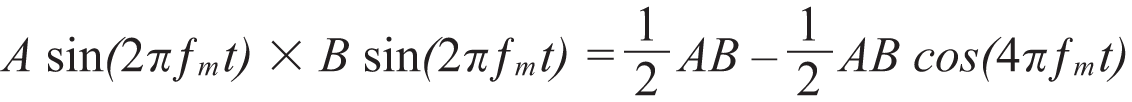(1)

The advantage of using this technique becomes evident when considering a noisy input signal. The output of the multiplication results in only the signal at the modulation frequency moving back to dc, with all other frequency components moving to other, nonzero frequencies. Figure 3 shows a system with strong noise sources at 50 Hz and 2.5 kHz. A very weak signal of interest is modulated with a 1-kHz sine wave. The result of multiplying the input with the reference is a signal at dc, and other signals at 950 Hz, 1050 Hz, 1.5 kHz, 2 kHz, and 3.5 kHz. The dc signal contains the desired information, so a low-pass filter can remove the other frequencies.Figure 3. Synchronous demodulation picks out a weak signal at 1 kHz in the presence of strong noise sources at 50 Hz and 2.5 kHz.

Any noise components that are close to the signal of interest will appear at frequencies close to dc so it is important to pick a modulation frequency that does not have strong noise sources near it. If this is not possible, a low-pass filter with a very low cutoff frequency and sharp response, at the expense of long settling time, will be required.

### Practical Lock-In Implementations

Generating a sine wave to modulate the signal source can be impractical, so some systems use a square wave instead. Generating a square-wave excitation is much simpler than generating a sine wave, because it can be done with some-thing as simple as a microcontroller pin that toggles an analog switch or a MOSFET.

Figure 4 shows an easy way to implement a lock-in amplifier. A microcontroller or other device generates a square wave excitation that causes the sensor to respond. The first amplifier would be a transimpedance amplifier for a photodiode, or an instrumentation amplifier for a strain gage.

The same signal that excites the sensor controls the ADG619 SPDT switch. When the excitation signal is positive, the amplifier is configured for a gain of +1. When the excitation is negative, the amplifier is configured for a gain of –1. This is mathematically equivalent to multiplying the measured signal by the reference square wave. The output RC filter removes signals at other frequencies, so the output voltage is a dc signal equal to half the peak-to-peak voltage of the measured square wave.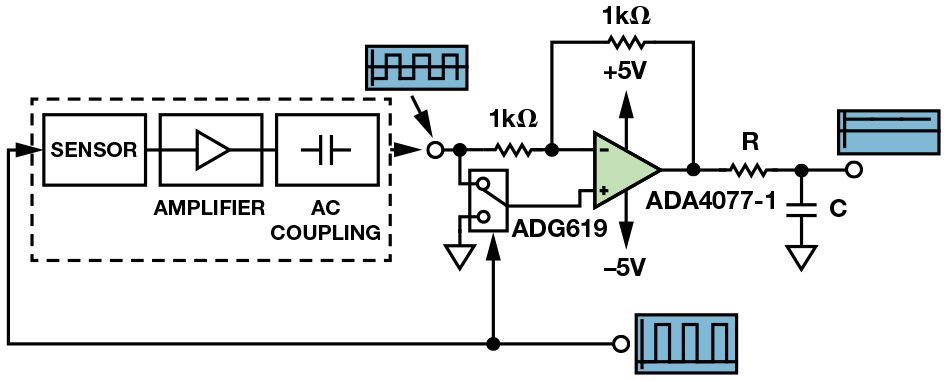Figure 4. Lock-in amplifier using square-wave excitation.

Although the circuit is simple, choosing the right op amp is important. The ac-coupled input stage removes most of the low-frequency input noise, but will not remove the 1/f noise and offset error from the last amplifier. The ADA4077-1 precision amplifier has 250 nV p-p noise from 0.1 Hz to 10 Hz and 0.55 µV/°C offset drift, making it an ideal candidate for this application.

A square-wave-based lock-in amplifier is simple, but its noise rejection is inferior to those using sine waves. Figure 5 shows the frequency domain representation of square wave excitation and reference signals. A square wave comprises an infinite sum of sine waves at the fundamental and all odd harmonics. Multiplying two square waves of the same frequency involves multiplying each sine component of the reference times each sine component of the measured signal. The result is a dc signal that contains energy from every harmonic of the square wave. Unwanted signals that appear at odd harmonic frequencies will not be filtered out, although they will be scaled down depending on the harmonic they fall in. Thus, it is important to choose a modulation frequency whose harmonics are not at the frequency or harmonic of any known noise sources. To reject line noise, for example, choose a modulation frequency of 1.0375 kHz, which does not coincide with harmonics of 50 Hz or 60 Hz, instead of using 1 kHz, which is the 20th harmonic of 50 Hz.

Even with this drawback, the circuit is simple and low cost. Using a low-noise amplifier and choosing the right modulation frequency can still result in a great improvement over trying to make a dc measurement.Figure 5. If the input signal (A) and the reference (B) are both square waves, multiplying them together (C) effectively demodulates every harmonic of the input signal.

### A Simple, Integrated Alternative

The circuit shown in Figure 4 requires an op amp, a switch, and some discrete components, plus a reference clock from a microcontroller. An alternative is to use an integrated synchronous demodulator, as shown in Figure 6. The ADA2200 includes a buffered input, a programmable IIR filter, a multiplier, and a block that shifts the reference signal by 90°, making it easy to measure or compensate for phase shifts between the reference clock and the input signal.Figure 6. ADA2200 block diagram.

Implementing a lock-in detection circuit with the ADA2200 simply requires applying a clock frequency that is 64× the desired reference frequency, as shown in Figure 7. The default configuration of the programmable filter is a band-pass response, removing the need to ac couple the signal. The sampled analog output generates images around multiples of the sampling rate, so an RC filter followed by a Σ-∆ ADC can remove these images and measure only the demodulated dc component of the signal.Figure 7. Lock-in amplifier implementation with the ADA2200.

### Improving the Square-Wave Lock-In Circuit

Figure 8 shows an improvement to a square-wave modulated circuit. The sensor is excited with a square wave, but the measured signal is multiplied with a sine wave of the same frequency and phase. Now, only the signal content at the fundamental frequency will move to dc, while all of the other harmonics will move to nonzero frequencies. This makes it easy to use a low-pass filter to eliminate everything but the dc component of the measured signal.Figure 8. Using a sine wave as the reference signal prevents noise from demodulating to dc.

One additional difficulty is that any phase shift between the reference signal and the measured signal will produce a smaller output than if they were perfectly in phase. This can occur if the sensor signal conditioning circuit includes filters that introduce phase delay. With an analog lock-in amplifier, the only way to address this is to include additional phase-compensation circuits in the reference signal path. This is not trivial, because the circuit must be adjustable to compensate for different phase delays, and will vary with temperature and component tolerance. An easier alternative is to add a second stage that multiplies the measured signal with a 90° phase-shifted version of the reference. The result of this second stage will be a signal proportional to the out-of-phase component of the input, as shown in Figure 9.

The outputs of the low-pass filters after the two multiplier stages are low-frequency signals proportional to the in-phase (I) and quadrature (Q) components of the input. To calculate the magnitude of the input signal, simply take sum of the squares of the I and Q outputs. An additional benefit of this architecture is that it allows the phase between the excitation/reference signal and the input to be calculated.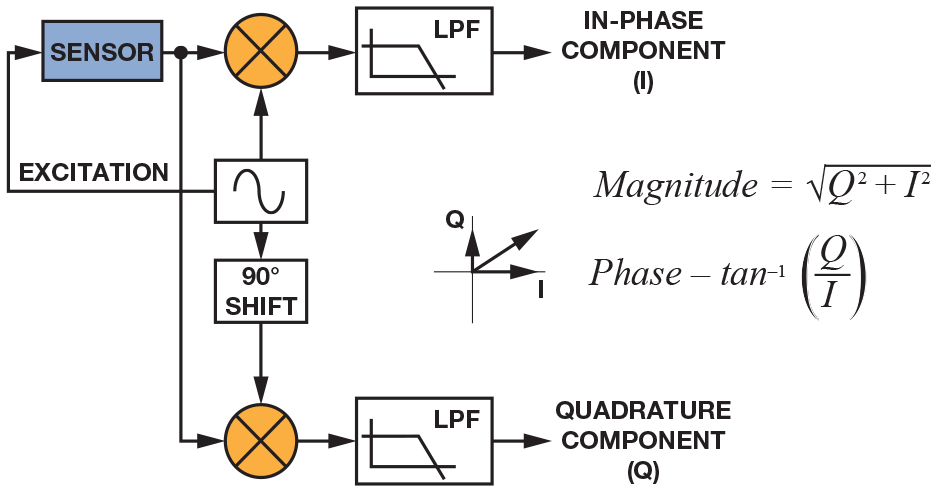Figure 9. Using a quadrature version of the reference signal to calculate magnitude and phase.

All of the lock-in amplifiers discussed so far generate a reference signal that excites a sensor. One final refinement is to allow an external signal to act as a reference. For example, Figure 10 shows a system that uses a broadband incandescent light to test the optical properties of a surface. A system like this can measure parameters such as the reflectivity of mirrors or the amount of contamination on a surface. It is much simpler to use a mechanical chopper disk to modulate an incandescent source than to apply electronic modulation. A low-cost position sensor near the chopper disk generates a square-wave reference signal to feed the lock-in amplifier. Rather than using this signal directly, a phase-locked loop generates a sine wave of the same frequency and phase as the input reference. One caveat of this approach is that the internally generated sine wave must have low distortion.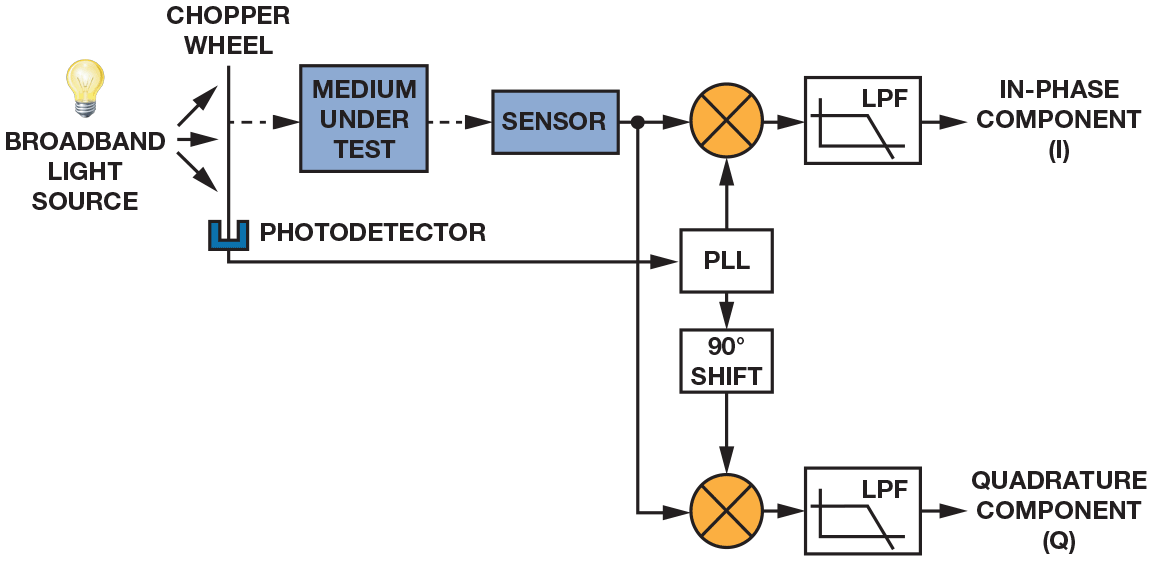Figure 10. Using a PLL to lock on to an external reference signal.

Although this system could be implemented using a discrete PLL and multipliers, using an FPGA to implement the lock-in amplifier function results in several performance benefits. Figure 11 shows a lock-in amplifier built with an FPGA using a front end based on the ADA4528-1 zero-drift amplifier and an AD7175 24-bit Σ-∆ ADC. This application does not need very high bandwidth, so the lock-in amplifier’s equivalent noise bandwidth can be set to 50 Hz. The device under test is any sensor that can be excited externally. The amplifier is configured with a noise gain of 20 to take advantage of the full-scale range of the ADC. Although dc errors do not affect the measurement, it is important to minimize offset drift and 1/f noise because they will decrease the available dynamic range, especially when the amplifier is configured for high gain.

The ADA4528-1’s 2.5 µV max input offset error represents only 10 ppm of the AD7175’s full scale input range with a 2.5-V reference. The digital high-pass filter after the ADC removes any dc offset and low-frequency noise. To calculate the output noise, first calculate the voltage noise density of the AD7175. The data sheet specifies the noise at 5.9 µV rms with an output data rate of 50 kSPS, using the sinc5 + sinc1 filter and with the input buffer enabled. The equivalent noise bandwidth with these settings is 21.7 kHz, which results in a voltage noise density of 40 nV/√Hz.

The 5.9-nV/√Hz broadband input noise of the ADA4528 appears at the output as 118 nV√Hz, resulting in a combined noise density of 125 nV/√Hz. Since the digital filter has an equivalent noise bandwidth of only 50 Hz, the output noise is 881 nV rms. With a ±2.5 V input range, this results in a system with 126-dB dynamic range. Bandwidth can be traded for dynamic range by adjusting the frequency response of the low-pass filter. For example, setting the filters for a bandwidth of 1 Hz results in 143-dB dynamic range, and setting the bandwidth to 250 Hz results in 119-dB dynamic range.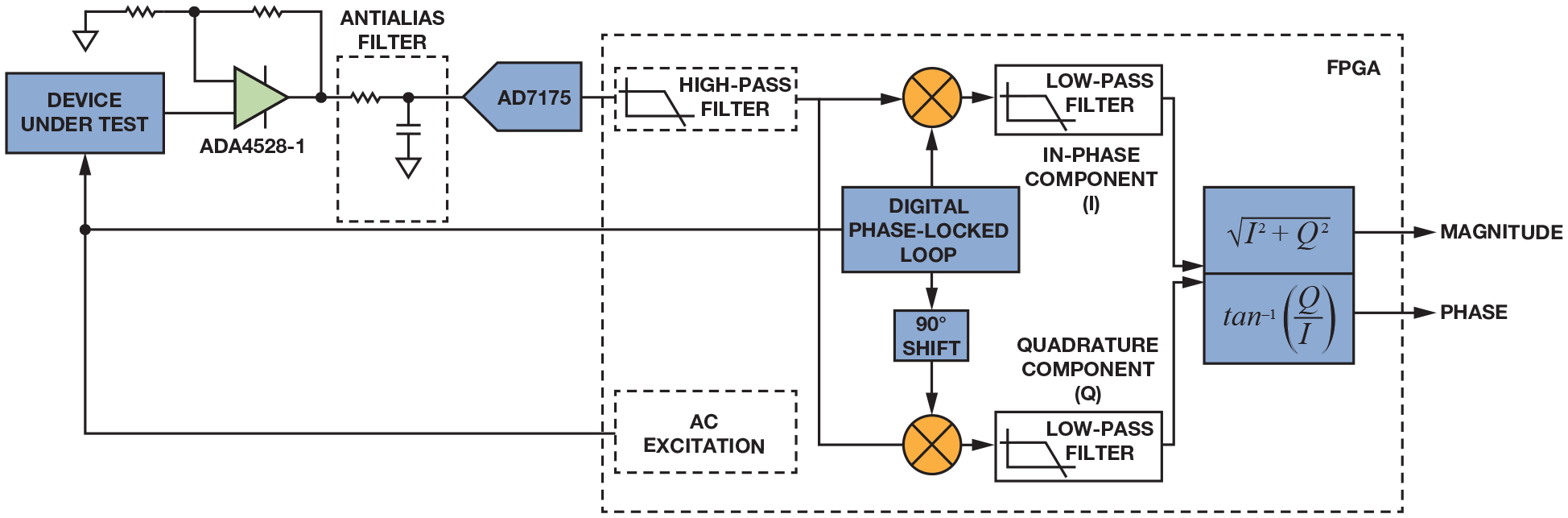Figure 11. FPGA-based lock-in amplifier.

The digital phase-locked loop generates a sine wave locked to the excitation signal, which can be internally or externally generated, and does not have to be a sine wave. Any harmonics in the reference sine wave will multiply with the input signal, demodulating noise and other unwanted signals present at the harmonic frequencies, just like when two square waves are multiplied. One advantage of digitally generating the reference sine wave is that very low distortion can be obtained by adjusting the numeric precision.

Figure 12 shows four digitally generated sine waves using 4-, 8-, 16-, and 32-bit precision. Obviously, using 4-bit precision results in performance not much different from that of Figure 5, but the situation quickly improves by using higher precision. With 16-bit precision, generating an analog signal with such low total harmonic distortion (THD) would be difficult, and with 32 bits, the THD is over –200 dB, making it impossible to match with an analog circuit. In addition, these are digitally generated signals, so they are perfectly repeatable. Once the data has been converted to digital and enters the FPGA, no additional noise or drift will be added.

After the multipliers, the low-pass filters remove any high- frequency components and output the in-phase and quadrature components of the signal. With an equivalent noise bandwidth of only 50 Hz, there is no reason to deliver data at the original sampling rate of 250 kSPS. The low-pass filters can include a decimation stage to reduce the output data rate. The last step is to calculate the magnitude and phase of the input signal from the in-phase and quadrature components.Figure 12. Numerically generated sine waves with different numeric precision.

### Conclusion

Small low-frequency signals buried in the noise floor can be difficult to measure, but applying modulation and lock-in amplifier techniques can provide high-precision measurements. In its simplest form, a lock-in amplifier can be an op amp that switches between two gains. Although this does not result in the lowest noise performance, the simplicity and low cost of the circuit makes it attractive when compared with a simple dc measurement. An improvement on this circuit is to use a sine wave reference and a multiplier, but this can be challenging to implement in the analog domain. For the ultimate performance, consider using a low-noise, high-resolution Σ-∆ ADC to digitize the input signal, generating the reference sine wave and all of the other elements in the digital domain.